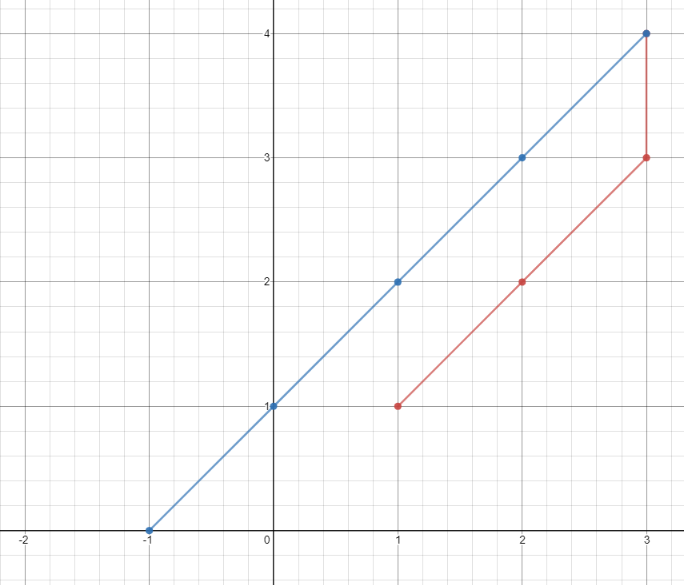1266. Minimum Time Visiting All Points

On a 2D plane, there are `n` points with integer coordinates `points[i] = [xi, yi]`. Return the minimum time in seconds to visit all the points in the order given by `points`.

You can move according to these rules:

• In `1` second, you can either:
• move vertically by one unit,
• move horizontally by one unit, or
• move diagonally `sqrt(2)` units (in other words, move one unit vertically then one unit horizontally in `1` second).
• You have to visit the points in the same order as they appear in the array.
• You are allowed to pass through points that appear later in the order, but these do not count as visits.

Example 1:```Input: points = [[1,1],[3,4],[-1,0]]
Output: 7
Explanation: One optimal path is [1,1] -> [2,2] -> [3,3] -> [3,4] -> [2,3] -> [1,2] -> [0,1] -> [-1,0]
Time from [1,1] to [3,4] = 3 seconds
Time from [3,4] to [-1,0] = 4 seconds
Total time = 7 seconds```

Example 2:

```Input: points = [[3,2],[-2,2]]
Output: 5
```

Constraints:

• `points.length == n`
• `1 <= n <= 100`
• `points[i].length == 2`
• `-1000 <= points[i], points[i] <= 1000`

1266. Minimum Time Visiting All Points
``````struct Solution;

impl Solution {
fn min_time_to_visit_all_points(points: Vec<Vec<i32>>) -> i32 {
let mut res = 0;
let n = points.len();
for i in 1..n {
let x1 = points[i - 1];
let y1 = points[i - 1];
let x2 = points[i];
let y2 = points[i];
res += i32::max((x2 - x1).abs(), (y2 - y1).abs());
}
res
}
}

#[test]
fn test() {
let points = vec_vec_i32![[1, 1], [3, 4], [-1, 0]];
let res = 7;
assert_eq!(Solution::min_time_to_visit_all_points(points), res);
}
``````